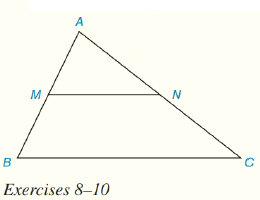Chapter 4.CT, Problem 8CTElementary Geometry For College St...

7th Edition
Alexander + 2 others
ISBN: 9781337614085

Solutions

Chapter
SectionElementary Geometry For College St...

7th Edition
Alexander + 2 others
ISBN: 9781337614085
Textbook Problem

In Δ A B C , M is the midpoint of A B ¯ and N is the midpoint if A C ¯ .a) How are the line segments M N ¯   and  B C ¯ related?b) Use an equation to state how the lengths MN and BC are related.To determine

(a)

To Find:

MN¯and BC¯ are related.

Explanation

Given:

M is the midpoint of AB¯ and N is the midpoint of AC¯.

Approach:

Since, M is the midpoint of AB¯ and N is the midpoint of AC¯

To determine

(b)

To Find:

The equation to show the relation between MN and BC.

Still sussing out bartleby?

Check out a sample textbook solution.

See a sample solution

The Solution to Your Study Problems

Bartleby provides explanations to thousands of textbook problems written by our experts, many with advanced degrees!

Get Started

Find the mean for the following set of scores: 2, 7, 9, 4, 5, 3, 0, 6

Essentials of Statistics for The Behavioral Sciences (MindTap Course List)

Simplify: 3656

Elementary Technical Mathematics

Simplify each expression. +6

Trigonometry (MindTap Course List)

True or False: converges mean exists.

Study Guide for Stewart's Multivariable Calculus, 8th

Which is the graph of ?

Study Guide for Stewart's Single Variable Calculus: Early Transcendentals, 8th